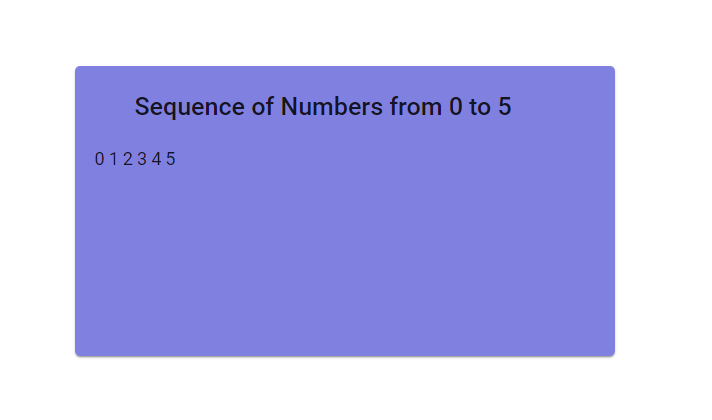# How to repeat HTML element multiple times using ngFor based on a number?

• Last Updated : 24 Jun, 2020

In Angular ngFor is directive which accomplishes the motive of displaying repeated or list of contents with least lines of code, this serves the same purpose as for loops in conventional programming languages.

We can print repeated lines of content based on a number using the javascript/typescript function Array() which will generate a list of number from 0 to n-1. We traverse this list to produce n repeated lines of content.

Example 1:
Demo.Component.ts

 `import { Component, OnInit } from ``'@angular/core'``; ` ` `  `@Component({ ` `  ``selector: ``'app-demo'``, ` `  ``templateUrl: ``'./demo.component.html'``, ` `  ``styleUrls: [``'./demo.component.css'``] ` `}) ` ` `  `export class DemoComponent implements OnInit { ` ` `  `  ``constructor() { ` `  ``} ` `  ``ngOnInit() { ` `  ``} ` `  ``//function to return list of numbers from 0 to n-1 ` `  ``numSequence(n: number): Array { ` `    ``return` `Array(n); ` `  ``} ` `} `

Demo.Component.html

 `<``mat-card` `class``=``"example-card"` `> ` `  ``<``mat-card-header``> ` `   ``<``h2` `>Sequence of Numbers from 0 to 5 ` `  `` ` `  ``<``mat-card-content``> ` `     ``` `    ``<``span` `*ngFor="let n of numSequence(6); ` `           ``let ``i` `= ``index``;">{{i}} ` `  `` ` ` `

Output:Example 2:
Inserting template in typescript file and repeating the same element 6 times.
Demo2.component.ts

 `import { Component, OnInit } from ``'@angular/core'``; ` ` `  `@Component({ ` `  ``selector: ``'app-demo'``, ` `  ``//template encapsulated within the component ts file ` `  ``// instead of separate template(html) ` `  ``template: ``' ` `  `` ` `  `` ` `   ``

Repeated GeeksforGeeks ` `  `` ` `  `` ` `    ``
` `      ``  ` `     ``
• ` `                ``GeeksforGeeks
• ` `    ``
` `  ``
` ` ` `'``, ` `  ``styleUrls: [``'./demo.component.css'``] ` `}) ` ` `  `export class DemoComponent implements OnInit { ` `  ``constructor() { ` `  ``} ` ` `  `  ``ngOnInit() { ` `  ``} ` `  `  `  ``//function to return list of numbers from 0 to n-1 ` `  ``numSequence(n: number): Array { ` `    ``return` `Array(n); ` `  ``} ` `} `

Output:My Personal Notes arrow_drop_up
Recommended Articles
Page :